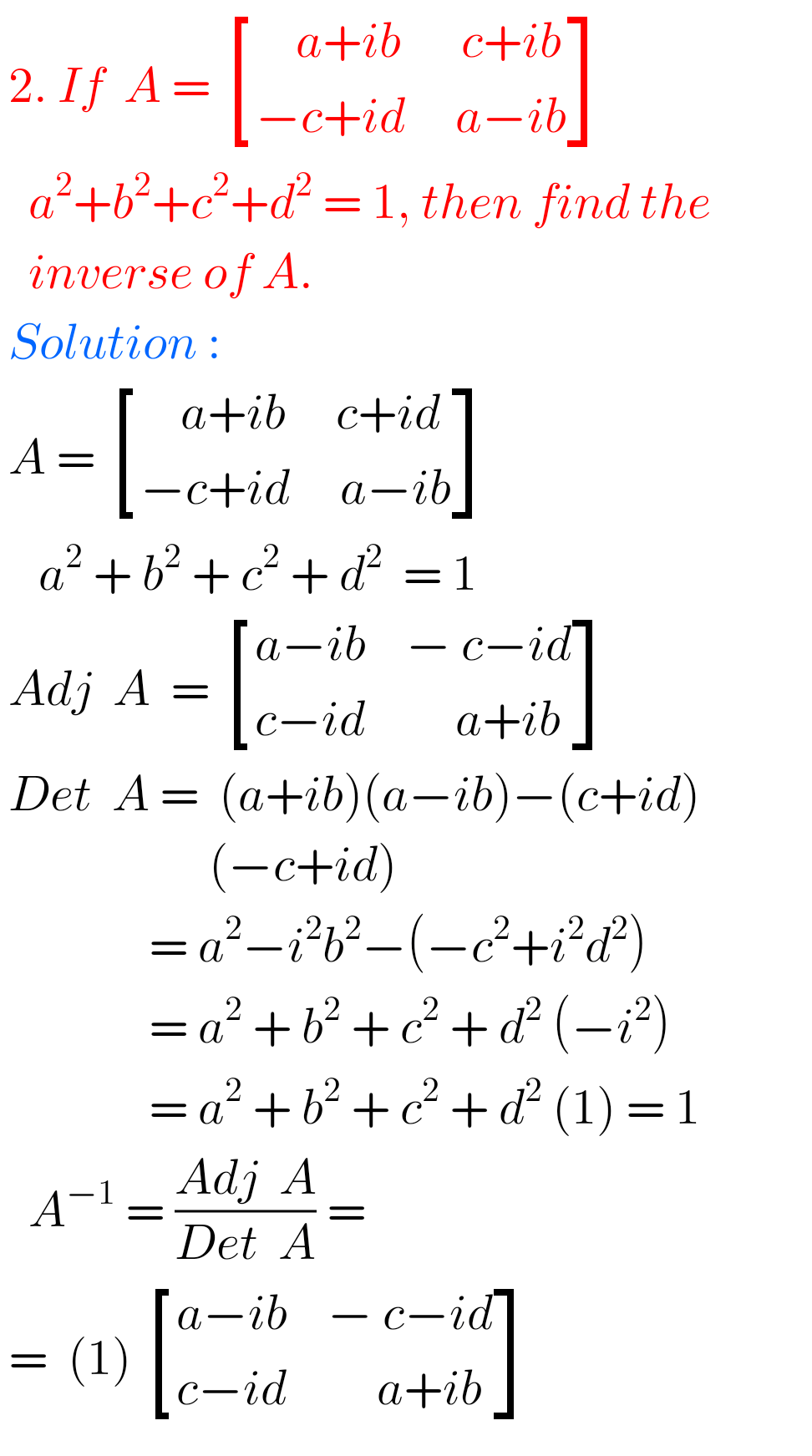# Matrix Problems with solutions Inter Maths 1A.## Intermediate Maths Solutions for Matrices Exercise 3(g)

Intermediate Maths solutions for Matrices Exercise 3(g) Inter Maths 1A text book Exercise 3(g) Matrices solutions are given. These solutions are very easy to understand. Please study the text book lesson Matrices very well. Observe the example problems and solutions given in the text book. Try them well. Observe the given solutions and try them …## Intermediate Maths Solutions for Matrices Exercise 3(f)

Intermediate Maths solutions for Matrices Exercise 3(f) Inter Maths 1A text book Exercise 3(f) Matrices solutions are given. These solutions are very easy to understand. Please study the text book lesson Matrices very well. Observe the example problems and solutions given in the text book. Observe the given solutions and try them in your own …## Intermediate Maths Solutions for Exercise 3(e) Matrices

Intermediate Maths solutions for Matrices Exercise 3(e) Inter Maths 1A text book Exercise 3(e) Matrices solutions are given. These solutions are very easy to understand. Please study the text book lesson Matrices very well. Observe the example problems and solutions given in the text book. Observe the given solutions and try them in your own …